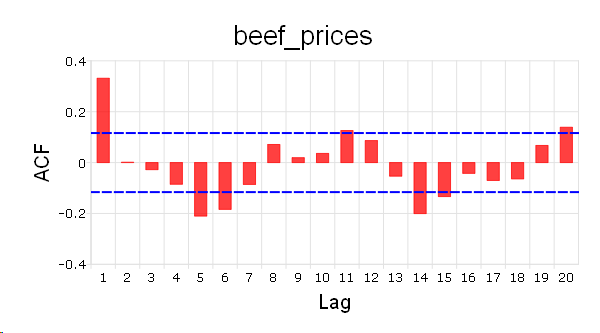# plotACF¶

## Purpose¶

Plot the autocorrelations function (ACF).

## Format¶

plotACF([myPlot, ]x, k, d)
Parameters
• myPlot (struct) – Optional argument, a plotControl structure for acf

• x (Nx1 vector) – data.

• k (scalar) – maximum number of autocorrelations to compute.

• d (scalar) – order of differencing.

## Examples¶

// Clear out variables in GAUSS workspace
new;

// Create file name with full path
file = getGAUSSHome() \$+ "examples/beef_prices.csv";

// Import dataset starting with row 2 and column 2

// Max lags
k = 20;

// Order of differencing
d = 1;

// ACF plot
struct plotControl mp;
mp = plotGetDefaults("bar");

plotSetTitle(&mp, "beef_prices", "Arial", 20);

plotACF(mp, x, k, d);


The plot is:## Remarks¶

To control the color and texture of the bars as well as whether they are stacked or side by side:

If you are passing a plotControl structure to your graph, you may use the function plotGetDefaults() and plotSetBar().

If you are not passing a plotControl structure, these properties are set in the Preferences. To access the Graphics Preferences, select Tools > Preferences from the GAUSS main menu. Select Graphics on the left side of the preferences and then select Bar from the list of graph types.